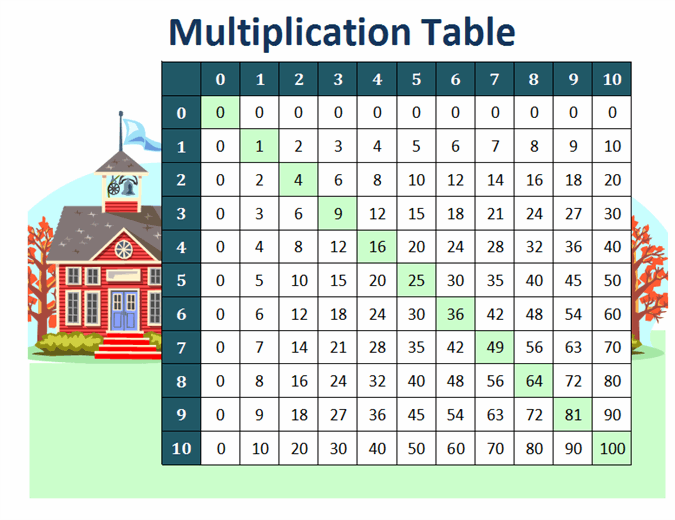Simplify the complex with data types in Excel. DOWNLOAD THE TEMPLATES NOW.

# Multiplication table (numbers 1 to 10)

Teach your students or children simple mathematics with this kid-friendly multiplication table. The multiplication table template lays out numbers from 1 through 10 and has a schoolhouse background image. This is an accessible math table template to teach mathematics at a basic level.

Excel